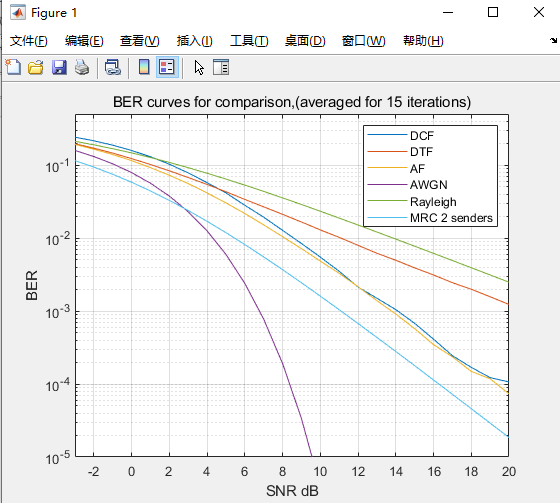2181264433

QQ ：2181264433MATLAB代做-FPGA代做-MRC误码率的matlab仿真

# MRC误码率的matlab仿真

### 2.部分程序：

%Comparison of Decode and F,Detect and F and Amplify and Forward
%BPSK in flat fading with WGN:
%plot simulation results of BER and theoratical results for it.
%combining the two signals using MRC
%using averaged BER

%%%%%%%%%house hold instuctions%%%%%%%%%%%%%%%%%%
clc
clear all
close all

sum_dcf = 0;
sum_dtf = 0;
sum_af = 0;
%%%%%%%%%%%%%%%%%%%User1:Source%%%%%%%%%%%%%%
N_bits = 15000; %Number of data bits

%number of iterations over which the results are going to be averaged
N_iter = 15;
for iter = 1:N_iter

data = round(rand(N_bits,1));%random data bits
%channel coding using rate 1/2 convolutional code:
trellis = poly2trellis(3,[5 7]); %trellis structure
c_data = convenc(data,trellis);

%BPSK modulation
tx = 2*c_data - 1;

%%%%%%%%%Channel characteristics%%%%%%%%%%%%%%%%
SNRdB = -3:20; %Range of SNR for which BER is investigated.

%additive noise and channel response for the relay channel:
noise_d = 1/sqrt(2) * (randn(2 * N_bits,1) + j * randn(2 * N_bits,1));
h_d = 1/sqrt(2) * (randn(2 * N_bits,1) + j * randn(2 * N_bits,1));

%interuser channel:
noise_r1 = 1/sqrt(2) * (randn(2 * N_bits,1) + j * randn(2 * N_bits,1));
h_r1 = 1/sqrt(2) * (randn(2 * N_bits,1) + j * randn(2 * N_bits,1));

noise_r2 = 1/sqrt(2) * (randn(2 * N_bits,1) + j * randn(2 * N_bits,1));
h_r2 = 1/sqrt(2) * (randn(2 * N_bits,1) + j * randn(2 * N_bits,1));

for k = 1:length(SNRdB)

SNR = 10^(SNRdB(k)/10); %convert SNRdB to linear value SNR

ftx_r1 = sqrt(SNR) * h_r1 .* tx + noise_r1;

%%%%%%%%%%%%%%%%% At the Relay %%%%%%%%%%%%%
%%%%%%%%%%%%%%%%%%%%%%%%%Decode n F%%%%%%
%equalizing at relay
eq_rx1 = ftx_r1 .* conj(h_r1);
%hard decision and converting from bipolar to bits
r_bits = (sign(real(eq_rx1)) + 1)/2;
%channel decoding:
dec_dcf_r1 = vitdec(r_bits,trellis,3,'term','hard');
%re-encoding using the same procedure as Source:
c_data2 = convenc(dec_dcf_r1,trellis);
%BPSK signal for the relay coded data:
tx2_dcf = 2 * c_data2 - 1;
%%%%%%%%%%%%%%%%%%%%%%%%%%%%%%%%%%%%%

%%%%%%%%%%%%%%%%%%Detect n F%%%

dec_dtf_r1 = sign(real(eq_rx1));
tx2_dtf = dec_dtf_r1;

%%%%%%%%%%%%%%%%%%%%%%%%%%%%%Apmlify n F%%

beta = sqrt(1./((SNR * abs(h_r1).^2) + 1));
%amplification:
ftx_amp = ftx_r1 .* beta;

%%%%%%%%%%%%%%%%%%%%%%%%%%Relay to Destination%
%DCF
ftx_dcf_r2 = sqrt(SNR) * tx2_dcf .* h_r2 + noise_r2 ;
%DTF
ftx_dtf_r2 = sqrt(SNR) * tx2_dtf .* h_r2 + noise_r2 ;
%AF
ftx_af_r2 = sqrt(SNR) * ftx_amp .* h_r2 + noise_r2 ;

%%%%%%%%%%%%%%%%% At the Destination%%%%%%%%%%%
ftx_d = sqrt(SNR)* tx .* h_d + noise_d;

%%%%%%%%%%%%%%%%%%%%%%%%DCF%%%%%%%%%%%
%%%%%%%%%%%%%%%%%%%MRC%%%%%%%%%%%%%%%
R_dcf = ftx_dcf_r2 .* conj(h_r2) + ftx_d .* conj(h_d);
%hard decisioning
dec_com_dcf = sign(real(R_dcf));

%%%%%%%%%%%BER calculations%%%%%%%%%%%%%%%%%%
%at destination:
err_com1(k) = sum(abs(dec_com_dcf - tx)/2);
simber_com1(k) = err_com1(k) / (2 * N_bits);

%%%%%%%%%%%%%%%%%%%%%%%%DTF%%%%%%%%%%%
%%%%%%%%%%%%%%%%%%%MRC%%%%%%%%%%%%%%%
R_dtf = ftx_dtf_r2 .* conj(h_r2) + ftx_d .* conj(h_d);
%hard decision
dec_com_dtf = sign(real(R_dtf));
%%%%%%%%%%%BER calculations%%%%%%%%%%%%%%%%%%
%at destination:
err_com2(k) = sum(abs(dec_com_dtf - tx)/2);
simber_com2(k) = err_com2(k) / (2 * N_bits);

%%%%%%%%%%%%%%%%%%%%%%%%AF%%%%%%%%%%%
%%%%%%%%%%%%%%%%%%%MRC%%%%%%%%%%%%%%%
R_af = ftx_af_r2 .* conj(h_r2) .* conj(h_r1) + ftx_d .* conj(h_d);
dec_com_af = sign(real(R_af));
%%%%%%%%%%%BER calculations%%%%%%%%%%%%%%%%%%
%at destination:
err_com3(k) = sum(abs(dec_com_af - tx)/2);
simber_com3(k) = err_com3(k) / (2 * N_bits);

theberawgn(k) = 0.5 * erfc (sqrt(SNR));%theoratical BER for AWGN
theberrayleigh(k) = 0.5 * (1 - sqrt(SNR./(1 + SNR))); %theoratical rayleigh

mue = sqrt(SNR/(1+SNR));
mrcth(k) = 0.25 * (2 + mue) * (1-mue)^2;%theoratical 2Rx MRC

end

sum_dcf = sum_dcf + simber_com1;
sum_dtf = sum_dtf + simber_com2;
sum_af = sum_af + simber_com3;
end

%average BER:
avgber_dcf = sum_dcf/N_iter;
avgber_dtf = sum_dtf/N_iter;
avgber_af = sum_af/N_iter;

%%%%%%%%%%%%%%%%%%%%%%%%%%%%%%%%%%%%%%%
%%%%%%%%%BER plots%%%%%%%%%%%%%%%%%%%%%%%%%%
%%%%%%%%%%%%%%%%%%%%%%%%%%%%%%%%%%%%%%%

figure
semilogy(SNRdB,avgber_dcf,SNRdB,avgber_dtf,SNRdB,avgber_af,SNRdB,theberawgn,SNRdB,theberrayleigh,SNRdB,mrcth);
axis([SNRdB(1) max(SNRdB) 10^-5 0.5]);
grid on
legend('DCF','DTF','AF','AWGN','Rayleigh','MRC 2 senders');
xlabel('SNR dB');
ylabel('BER');
title(['BER curves for comparison,(averaged for ',num2str(N_iter),' iterations)']);

### 3.仿真结论：